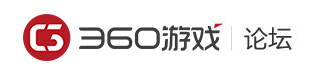《群雄风云》兵种介绍

# 《群雄风云》兵种介绍[复制链接][复制全部]

【入伍新卒】攻击=6；生命=30；实力等级=1；单体攻击
【戚家军】攻击=7；生命=88；实力等级=13；
【北府兵】攻击=21；生命=260；实力等级=25；
【陷阵营】攻击=44；生命=530；实力等级=37；
【秦国锐士】攻击=110；生命=1300；实力等级=58；
【齐国技击】攻击=127；生命=1800；实力等级=60；
【魏国武卒】攻击=145；生命=1500；实力等级=60；

【新兵弓手】攻击=11；生命=17；实力等级=4；
【精英弓手】攻击=40；生命=60；实力等级=16；
【英勇骑兵】攻击=100；生命=160；实力等级=28；
【南蛮骑兵】攻击=210；生命=310；实力等级=40；
【辽军射手】攻击=460；生命=650；实力等级=58；
【西凉神射营】攻击=555；生命=860；实力等级=60；
【诸葛连弩营】攻击=600；生命=760；实力等级=60；

【新手弓兵】攻击=10；生命=30；实力等级=7；无穿透能力
【轻甲弓兵】攻击=28；生命=110；实力等级=19；
【重甲铁骑】攻击=68；生命=250；实力等级=31；
【猛虎铁骑】攻击=130；生命=470；实力等级=43；
【西凉铁骑】攻击=260；生命=850；实力等级=58；
【蒙古铁骑】攻击=290；生命=1200；实力等级=60；
【玄甲精骑】攻击=345；生命=960；实力等级=60；

【轻型投石车】攻击=5；生命=38；实力等级=10；
【中型投石车】攻击=16；生命=120；实力等级=22；
【重型投石车】攻击=37；生命=270；实力等级=34；
【巨型投石车】攻击=67；生命=480；实力等级=46；
【攻城投石车】攻击=125；生命=800；实力等级=58；
【西域回回炮】攻击=150；生命=1050；实力等级=60；
【霹雳烈朴刀】攻击=170；生命=1100；实力等级=60；"

【步兵】加全体友军伤害
【骑兵】加全体友军暴击
【弓兵】减少受到的伤害
【攻城器械】减少受到的投石伤害LV1到LV8的兵种,光环效果分别是5%,10%,15%,20%,25%,25%,30%,30%；同一只部队中相同类型的光环效果不可叠加。"

【步兵】对攻城器械造成125%的伤害
【骑兵】对步兵造成125%的伤害,对弓兵造成80%的伤害
【弓兵】对骑兵造成125%的伤害,对步兵造成80%的伤害
【攻城器械】对弓兵造成125%的伤害,对骑兵造成80%的伤害；"

 您需要登录后才可以回帖 登录 | 立即注册 回帖后跳转到最后一页